# A new way to BOW Analysis & Feature Engineering

One of my friends asked me a problem — “how can we compare the BOW across different categories or labels? Where categories or labels could be sentiment or state or customer loyalty.

My intuitive response was — to create a Bar graph of the frequency of words for each category. This really is a simple to implement solution, but have various drawbacks, some of which are:

• The data scientist/analyst working on this will be required to compare words and their frequencies across all the categories, which in some cases like countries or could easily get over 100
• Comparing the frequencies may not give any insights. For example, we have word — “data” and its frequency across label1 and label2 be 150 & 100 respectively. Yes, indeed there is a difference of 50 but is this difference even relevant?
• What if -I want to know what are the top words which differentiate these categories without building a model/classifier, is there even a way to tell that?

Then the next idea was to create a Word Cloud, but even it does not solve the above-mentioned problem.

After thinking for a while I knew that solution lies in comparing the frequencies across the categories, but could not find the answer in One-Hot Encoding or Count Vectorizing or TF-IDF as there is a common issue in using any of these.

The issue is these create features and their values, for each of the documents, and then how can we roll these up at the label/category level? Even let’s take the case of Count Vectorizer — we will get the frequencies of words present in each document — but to do any analysis at the label/category level we will be required to sum up the counts and roll up to category level. Once we roll up, we can definitely make the difference in frequencies of words across labels but again — it won’t solve the third issue mentioned above. That is if the difference of frequency of word ‘data’ is 50, what does it tell us? Is this even significant?

Now, you can guess where are we headed — we have differences in frequencies and we want to know if the differences are significant or not. With this comes the STATISTICS to our rescue.

I am assuming you are aware of the various tests like z-test, t-test, ANOVA, etc. We use these tests to compare multiple means or distributions, which aligns with the problem we are trying to solve here.

The below table contains the frequency of a word across the labels — Target-0 and Target-1., which is nothing but the distributions. We can easily use the tests like z-test, t-test, etc. and compare these distributions and if the difference turns out to be significant (given the significance level) we can say that such words have different distributions across the labels and hence, can be the distinguishing factors in the model.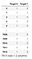Frequency of a word across the labels — Target0 & Target1

So, this way we can even use this technique for feature engineering and reduce the number of features in our models.

Easy right? Yes, indeed only after we have taken care of one last point.

The z-test, t-test, or ANCOVA are parametric tests, meaning they make an assumption about the data and one such main assumption is that the data to be normally distributed.

But, this assumption may or may not hold true for all the features/words in the dataset. So, we will have to rely on some Non-parametric tests which do not make any such assumptions.

And we have few such tests and the only we will use is — Mann Whitney U test. This test compares two distributions at a time and is ideal for the cases where we have Binomial labels and in case of Multinomial we can use — Kruskal-Wallis test.

Kruskal-Wallis test is similar to ANOVA which tells us whether the distribution across the labels is the same or not and it does not tell distributions of which labels differ and to get the same we can apply pair-wise Mann Whitney U test.

Let’s apply all the learning and for the same — Disaster Tweets Classification dataset from Kaggle has been used.

Read the dataset.

`# read the datasettrain_df = pd.read_csv(“/kaggle/input/nlp-getting-started/train.csv”)[[‘target’, ‘text’]]print(“Size of the data: “, train_df.shape)train_df.head(2)`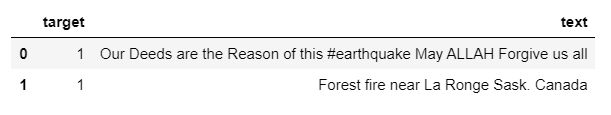A few sample records

Now, we will convert the sentences in our dataset to Bag of words using CrazyTokenizer. Also, some additional cleaning is done in the below preprocessor function.

This function will be passed to CountVectorizer which will give out the frequency of words in each of the records in the input dataset.

`# initializing the CrazyTokenizer to convert sentences into Bag of words featuresctokenizer = CrazyTokenizer(lowercase=True, normalize=1, remove_punct=True, ignore_stopwords=True, stem='lemm',                             remove_breaks=True, hashtags=' ', twitter_handles=' ', urls=' ')# testing on a sample textctokenizer.tokenize("There's an emergency evacuation happening now in the building across the street")`BOW of a sentence
`# the preprocessor function for CountVectorizerdef preprocessor(text):# split the sentence into tokens    tokens = ctokenizer.tokenize(text)# remove any numeric character    tokens = [re.sub(r'[0-9-]+', '', token) for token in tokens]# remove stop words or any token having size <3    tokens = [token for token in tokens if len(token) >= 3]     return " ".join(tokens)# create the CountVectorizer objectcvectorizer = CountVectorizer(preprocessor=preprocessor, min_df=20)# to get the featuresfeatures = cvectorizer.fit_transform(train_df['text'])# create a dataframe of featuresfeatures = pd.DataFrame(features.toarray(), columns=cvectorizer.get_feature_names())features.head(5)`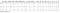Features created using CountVectorizer

let’s now also bring the target variable along with the features.

`# merge features with the reviews_data to get labelstrain_df_w_ft = pd.concat([train_df[['target']], features], axis=1)train_df_w_ft.head(5)`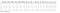Features data with Target variable

Now, we will be plotting a bar graph displaying the top 30 words belonging to each of the target values.

`# a generic function to plot bar-graphs and will be used throughout the analysisdef plot_bar_graph(xs, ys, names, xlabel, ylabel, title):# create figure object    fig = go.Figure()# create bar chart for each of the series provided     for (x, y), name in zip(zip(xs, ys), names):fig.add_trace(go.Bar(x=x, y=y, name=name, orientation='v'))# Here we modify the tickangle of the xaxis, resulting in rotated labels.    fig.update_layout(        barmode='group',        autosize=False,        width=1300,        height=500,        margin=dict(l=5, r=5, b=5, t=50, pad=5),        xaxis={'type': 'category', 'title': xlabel},        yaxis_title=ylabel,        title=title    )    fig.show()top_x = 30words_lists = []frequencies = []targets = []for target in train_df_w_ft['target'].unique():# add label name    targets.append("Target-{}".format(target))# get the top words    word_freq = train_df_w_ft[train_df_w_ft['target'] == target].iloc[:, 1:].sum(axis=0)    word_freq = sorted(word_freq.to_dict().items(), key=lambda x: x, reverse=True)[: top_x]# append the words    words_lists.append([x for x in word_freq])# append the frequencies    frequencies.append([x for x in word_freq])plot_bar_graph(words_lists, frequencies, targets, "Words", "Frequency", "Frequency of Words across Targets")`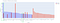Frequency of top 30 words across target values.

I have highlighted two words in the above chart — ‘fire’ & ‘emergency’. For the word ‘fire’ the difference in frequencies between the targets is huge (~180), while the difference is very very small in the case of the word ‘emergency’.

As we discussed above, our aim is to identify if these differences are even significant or not and this matters because both the words appeared in sentences across both the target values. We want to know does it make these features insignificant i.e. whether they have a say or not in deciding if a sentence belongs to Target-0 or Target-1.

Below is the result of the Mann-Whitney U test for the word — ‘fire’.

`fire_data0 = train_df_w_ft[train_df_w_ft['target'] == 0]['fire']fire_data1 = train_df_w_ft[train_df_w_ft['target'] == 1]['fire']mannwhitneyu(fire_data0, fire_data1)`

From the test we got the p-value of ~0 which is less than 0.05 (our significance level), hence we can conclude that the frequency distributions of the ‘fire’ are significantly different across the target values (with 95% confidence).

Let’s also look at the same for — ‘emergency’.

`emergency_data0 = train_df_w_ft[train_df_w_ft['target'] == 0]['emergency']emergency_data1 = train_df_w_ft[train_df_w_ft['target'] == 1]['emergency']mannwhitneyu(emergency_data0, emergency_data1)`

Here, the p-value is 0.07 which is greater than the significance level of 0.05, hence we can conclude that the frequency distributions of ‘emergency’ are similar with 95% confidence.

Now, its time for the desert — meaning let’s look at the words which have significantly different distributions across the targets, the problem we wanted to solve.

The below code will apply the Mann Whitney U test for all the words in the dataset and will keep a record of those words which had different distributions. Also, will be plotting such 50 words.

`words_significance = []for word in cvectorizer.get_feature_names():# get the pvalue    _, pval = mannwhitneyu(        train_df_w_ft[train_df_w_ft['target'] == 0][word],        train_df_w_ft[train_df_w_ft['target'] == 1][word]    )# check for significance    if pval < 0.05:        words_significance.append((word, pval))print("Total Number of words: ", len(cvectorizer.get_feature_names()))print("Number of words having different distributions with confidence of 95%: ", len(words_significance))# plot the top words by pvaluetop_x = 50# seperate the word and pvalueswords_list = [x for x in words_significance][: top_x]significance = [0.05 - x for x in words_significance][: top_x]# get the total frequencies of significantly different words across labelsfreq_label0 = [train_df_w_ft[train_df_w_ft['target'] == 0][x].sum() for x in words_significance]freq_label1 = [train_df_w_ft[train_df_w_ft['target'] == 1][x].sum() for x in words_significance]# plot the bar graphplot_bar_graph([words_list, words_list], [freq_label0, freq_label1], ['target0_freq', 'target1_freq'], "Words", "Frequency", "Frequency of Words across Targets")`

As we can observe from the above chart that there are words that appear in both the target values but the difference in frequencies is significant.

We are also left with only 451 words out of 633, which is like 71% of the original features and hence this can be used as one of the techniques for feature selection.

I also plotted the difference of 0.05 and the p-value of the above words. So, the words having y-axis value closer to 0 will indicate that their p-value was closer to the level of significance and hence comparatively will be less important than the ones whose value on the y-axis is closer to 0.05 as this indicates that their p-value was close to 0.

There are words like — ‘ago’, ‘big’, ‘blue’ having lower significance in comparison to others.

This is all for the analysis.

The code of the above analysis can be found on Kaggle and can be accessed via — https://www.kaggle.com/pikkupr/disaster-tweets-bow-analysis-across-targets

Hope you find my work above valuable and please do share your opinions about this methodology and if there are any suggestions to improve the same, please do comment as I would love to hear your thoughts.

Data Scientist | Mentor | Retail | Telecom | Social Media

## More from Prateek Jain

Data Scientist | Mentor | Retail | Telecom | Social Media